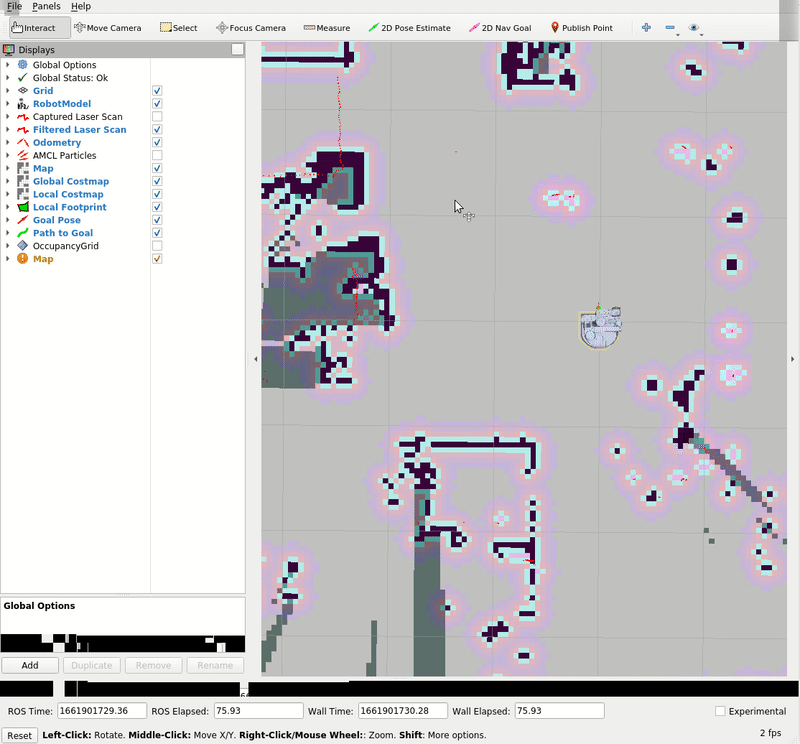# Example 13

In this example, we will be utilizing the move_base package, a component of the ROS navigation stack, to send base goals to the Stretch robot.

## Build a map

First, begin by building a map of the space the robot will be navigating in. If you need a refresher on how to do this, then check out the Navigation Stack tutorial.

## Getting Started

Next, with your created map, we can navigate the robot around the mapped space. Run:

``````roslaunch stretch_navigation navigation.launch map_yaml:=\${HELLO_FLEET_PATH}/maps/<map_name>.yaml
``````

Where `\${HELLO_FLEET_PATH}` is the path of the `<map_name>.yaml` file.

Note

It's likely that the robot's location on the map does not match the robot's location in real space. In the top bar of Rviz, use `2D Pose Estimate` to lay an arrow down roughly where the robot is located in real space. Below is a gif for reference.Now we are going to use a node to send a move_base goal half a meter in front of the map's origin. run the following command in a new terminal to execute the navigation.py node.

``````cd catkin_ws/src/stretch_tutorials/src/
``````

### The Code

``````#!/usr/bin/env python3

import rospy
import actionlib
import sys
from move_base_msgs.msg import MoveBaseAction, MoveBaseGoal
from geometry_msgs.msg import Quaternion
from tf import transformations

"""
A simple encapsulation of the navigation stack for a Stretch robot.
"""
def __init__(self):
"""
Create an instance of the simple navigation interface.
:param self: The self reference.
"""
self.client = actionlib.SimpleActionClient('move_base', MoveBaseAction)
self.client.wait_for_server()

self.goal = MoveBaseGoal()

self.goal.target_pose.pose.position.x = 0.0
self.goal.target_pose.pose.position.y = 0.0
self.goal.target_pose.pose.position.z = 0.0
self.goal.target_pose.pose.orientation.x = 0.0
self.goal.target_pose.pose.orientation.y = 0.0
self.goal.target_pose.pose.orientation.z = 0.0
self.goal.target_pose.pose.orientation.w = 1.0

def get_quaternion(self,theta):
"""
A function to build Quaternians from Euler angles. Since the Stretch only
rotates around z, we can zero out the other angles.
:param theta: The angle (radians) the robot makes with the x-axis.
"""
return Quaternion(*transformations.quaternion_from_euler(0.0, 0.0, theta))

def go_to(self, x, y, theta):
"""
Drive the robot to a particular pose on the map. The Stretch only needs
(x, y) coordinates and a heading.
:param x: x coordinate in the map frame.
:param y: y coordinate in the map frame.
:param theta: heading (angle with the x-axis in the map frame)
"""
x, y, theta))

self.goal.target_pose.pose.position.x = x
self.goal.target_pose.pose.position.y = y
self.goal.target_pose.pose.orientation = self.get_quaternion(theta)

self.client.send_goal(self.goal, done_cb=self.done_callback)
self.client.wait_for_result()

def done_callback(self, status, result):
"""
The done_callback function will be called when the joint action is complete.
:param self: The self reference.
:param status: status attribute from MoveBaseActionResult message.
:param result: result attribute from MoveBaseActionResult message.
"""
if status == actionlib.GoalStatus.SUCCEEDED:
rospy.loginfo('{0}: SUCCEEDED in reaching the goal.'.format(self.__class__.__name__))
else:
rospy.loginfo('{0}: FAILED in reaching the goal.'.format(self.__class__.__name__))

if __name__ == '__main__':
nav.go_to(0.5, 0.0, 0.0)
``````

### The Code Explained

Now let's break the code down.

``````#!/usr/bin/env python3
``````

Every Python ROS Node will have this declaration at the top. The first line makes sure your script is executed as a Python3 script.

``````import rospy
import actionlib
import sys
from move_base_msgs.msg import MoveBaseAction, MoveBaseGoal
from geometry_msgs.msg import Quaternion
from tf import transformations
``````

You need to import `rospy` if you are writing a ROS Node.

``````self.client = actionlib.SimpleActionClient('move_base', MoveBaseAction)
self.client.wait_for_server()
``````

Set up a client for the navigation action. On the Stretch, this is called `move_base`, and has type `MoveBaseAction`. Once we make the client, we wait for the server to be ready.

``````self.goal = MoveBaseGoal()
``````

Make a goal for the action. Specify the coordinate frame that we want, in this instance the `map` frame. Then we set the time to be now.

``````self.goal.target_pose.pose.position.x = 0.0
self.goal.target_pose.pose.position.y = 0.0
self.goal.target_pose.pose.position.z = 0.0
self.goal.target_pose.pose.orientation.x = 0.0
self.goal.target_pose.pose.orientation.y = 0.0
self.goal.target_pose.pose.orientation.z = 0.0
self.goal.target_pose.pose.orientation.w = 1.0
``````

Initialize a position in the coordinate frame.

``````def get_quaternion(self,theta):
"""
A function to build Quaternians from Euler angles. Since the Stretch only
rotates around z, we can zero out the other angles.
:param theta: The angle (radians) the robot makes with the x-axis.
"""
return Quaternion(*transformations.quaternion_from_euler(0.0, 0.0, theta))
``````

A function that transforms Euler angles to quaternions and returns those values.

``````def go_to(self, x, y, theta, wait=False):
"""
Drive the robot to a particular pose on the map. The Stretch only needs
(x, y) coordinates and a heading.
:param x: x coordinate in the map frame.
:param y: y coordinate in the map frame.
:param theta: heading (angle with the x-axis in the map frame)
"""
x, y, theta))
``````

The `go_to()` function takes in the 3 arguments, the x and y coordinates in the `map` frame, and the heading.

``````self.goal.target_pose.pose.position.x = x
self.goal.target_pose.pose.position.y = y
self.goal.target_pose.pose.orientation = self.get_quaternion(theta)
``````

The `MoveBaseGoal()` data structure has three goal positions (in meters), along each of the axes. For Stretch, it will only pay attention to the x and y coordinates, since it can't move in the z-direction.

``````self.client.send_goal(self.goal, done_cb=self.done_callback)
self.client.wait_for_result()
``````

Send the goal and include the `done_callback()` function in one of the arguments in `send_goal()`.

``````def done_callback(self, status, result):
"""
The done_callback function will be called when the joint action is complete.
:param self: The self reference.
:param status: status attribute from MoveBaseActionResult message.
:param result: result attribute from MoveBaseActionResult message.
"""
if status == actionlib.GoalStatus.SUCCEEDED:
rospy.loginfo('{0}: SUCCEEDED in reaching the goal.'.format(self.__class__.__name__))
else:
rospy.loginfo('{0}: FAILED in reaching the goal.'.format(self.__class__.__name__))
``````

Conditional statement to print whether the goal status in the `MoveBaseActionResult` succeeded or failed.

``````rospy.init_node('navigation', argv=sys.argv)
``````

The next line, `rospy.init_node(NAME, ...)`, is very important as it tells rospy the name of your node -- until rospy has this information, it cannot start communicating with the ROS Master.

Note

The name must be a base name, i.e. it cannot contain any slashes "/".

Declare the `StretchNavigation` object.

``````nav.go_to(0.5, 0.0, 0.0)
``````

Send a move base goal half a meter in front of the map's origin.